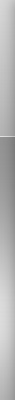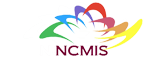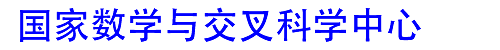网站地图 | 联系我们 | English | 意见反馈 | 主任信箱
 首页中心概况新闻动态科研进展交流合作人才培养研究队伍人才招聘政策规章数学交叉科学传播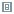科研进展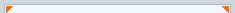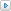科研成果研究专题获奖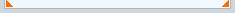现在位置：首页 > 科研进展 > 科研成果
 折扣型哈密顿-雅克比方程的粘性解负向极限 【大 中 小】【打印】【关闭】 2023-1-16Suppose M is a closed Riemannian manifold. For a $$C^2$$ generic (in the sense of Ma？é) Tonelli Hamiltonian $$H: T^*M\rightarrow \mathbb {R}$$, the minimal viscosity solution $$u_\lambda ^-:M\rightarrow \mathbb {R}$$ of the negative discounted equation \begin{aligned} -\lambda u+H(x,d_xu)=c(H),\quad x\in M,\ \lambda >0 \end{aligned} with the Ma？é’s critical value c(H) converges to a uniquely established viscosity solution $$u_0^-$$ of the critical Hamilton–Jacobi equation \begin{aligned} H(x,d_x u)=c(H),\quad x\in M \end{aligned} as $$\lambda \rightarrow 0_+$$. We also propose a dynamical interpretation of $$u_0^-$$.     Publication:Journal of Dynamics and Differential Equations (2022)https://doi.org/10.1007/s10884-022-10227-1　　 Author:Ya-Nan WangSchool of Mathematical Sciences, Nanjing Normal University, Nanjing, 210097, China　　 Jun YanSchool of Mathematical Sciences, Fudan University and Shanghai Key Laboratory for Contemporary Applied Mathematics, Shanghai, 200433, China　　 Jianlu ZhangHua Loo-Keng Key Laboratory of Mathematics & Mathematics Institute, Academy of Mathematics and Systems Science, Chinese Academy of Sciences, Beijing, 100190, ChinaEmail: jzhang87@amss.ac.cn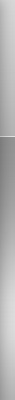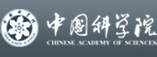欢迎访问国家数学与交叉科学中心　 地址：北京海淀区中关村东路55号　邮编：100190　电话: 86-10-62613242 Fax: 86-10-62616840 邮箱: ncmis@amss.ac.cn